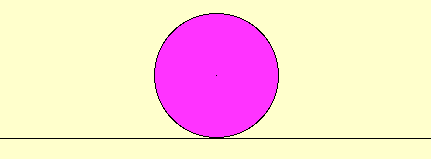Area of a Circle 2.

The formula for the area of a circle is a little more difficult and requires methods of successively better approximations.

The usual definition of π is the ratio of the circumference of a circle to its diameter d, so that the circumference of a circle is πd, or  2πr, where r is the radius.

The animation below shows that a circle can be divided into concentric rings which can be unrolled to closely resemble a triangle (with height  r and base 2πr) of area πr ².   By dividing the circle into more rings and unrolling, the result more closely matches a triangle, so the approximation becomes better.  Taking more and more rings, the triangles approximate the area of the circle arbitrarily closely and we conclude that the area of a circle is πr ².Rectangle :  :::: : :

Animations by Bettina Richmond and Tom Richmond.
Tom.Richmond@wku.edu
Updated May 22, 2008.